# Continuous Data and the Gaussian Distribution¶

### Example Problem¶

Consider a set of observations $D=\{x_1,…,x_N\}$ in the 2-dimensional plane (see Figure). All observations were generated by the same process. We now draw an extra observation $x_\bullet = (a,b)$ from the same data generating process. What is the probability that $x_\bullet$ lies within the shaded rectangle $S$?

In :
using Pkg;Pkg.activate("probprog/workspace/");Pkg.instantiate();
IJulia.clear_output();

In :
using Distributions, PyPlot
N = 100
generative_dist = MvNormal([0,1.], [0.8 0.5; 0.5 1.0])
function plotObservations(obs::Matrix)
plot(obs[1,:], obs[2,:], "kx", zorder=3)
fill_between([0., 2.], 1., 2., color="k", alpha=0.4, zorder=2) # Shaded area
text(2.05, 1.8, "S", fontsize=12)
xlim([-3,3]); ylim([-2,4]); xlabel("a"); ylabel("b")
end
D = rand(generative_dist, N) # Generate observations from generative_dist
plotObservations(D)
x_dot = rand(generative_dist) # Generate x∙
plot(x_dot, x_dot, "ro");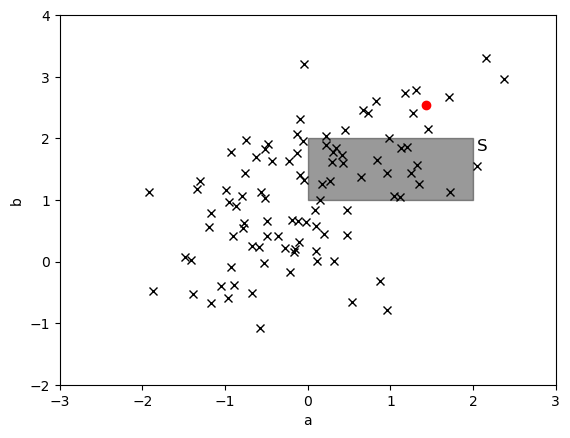### The Gaussian Distribution¶

• Consider a random (vector) variable $x \in \mathbb{R}^M$ that is "normally" (i.e., Gaussian) distributed. The moment parameterization of the Gaussian distribution is completely specified by its mean $\mu$ and variance $\Sigma$ and given by $$p(x | \mu, \Sigma) = \mathcal{N}(x|\mu,\Sigma) \triangleq \frac{1}{\sqrt{(2\pi)^M |\Sigma|}} \,\exp\left\{-\frac{1}{2}(x-\mu)^T \Sigma^{-1} (x-\mu) \right\}\,.$$ where $|\Sigma| \triangleq \mathrm{det}(\Sigma)$ is the determinant of $\Sigma$.
• Alternatively, the _canonical_ (a.k.a. _natural_ or _information_ ) parameterization of the Gaussian distribution is given by $$\begin{equation*} p(x | \eta, \Lambda) = \mathcal{N}_c(x|\eta,\Lambda) = \exp\left\{ a + \eta^T x - \frac{1}{2}x^T \Lambda x \right\}\,. \end{equation*}$$
• $a = -\frac{1}{2} \left( M \log(2 \pi) - \log |\Lambda| + \eta^T \Lambda \eta\right)$ is the normalizing constant that ensures that $\int p(x)\mathrm{d}x = 1$.
• $\Lambda = \Sigma^{-1}$ is called the precision matrix.
• $\eta = \Sigma^{-1} \mu$ is the natural mean or for clarity often called the precision-weighted mean.

### Why the Gaussian?¶

• Why is the Gaussian distribution so ubiquitously used in science and engineering? (see Jaynes, section 7.1.4).
• (1) Operations on probability distributions tend to lead to Gaussian distributions:
• Any smooth function with single rounded maximum, if raised to higher and higher powers, goes into a Gaussian function. (Example: sequential Bayesian inference).
• The Gaussian distribution has higher entropy than any other with the same variance.
• Therefore any operation on a probability distribution that discards information but preserves variance gets us closer to a Gaussian.
• As an example, see Jaynes, section 7.1.4 for how this leads to the Central Limit Theorem, which results from performing convolution operations on distributions.
• (2) Once the Gaussian has been attained, this form tends to be preserved. e.g.,
• The convolution of two Gaussian functions is another Gaussian function (useful in sum of 2 variables and linear transformations)
• The product of two Gaussian functions is another Gaussian function (useful in Bayes rule).
• The Fourier transform of a Gaussian function is another Gaussian function.

### Transformations and Sums of Gaussian Variables¶

• A linear transformation $z=Ax+b$ of a Gaussian variable $\mathcal{N}(x|\mu_x,\Sigma_x)$ is Gaussian distributed as $$p(z) = \mathcal{N} \left(z \,|\, A\mu_x+b, A\Sigma_x A^T \right) \tag{SRG-4a}$$
• In fact, after a linear transformation $z=Ax+b$, no matter how $x$ is distributed, the mean and variance of $z$ are always given by $\mu_z = A\mu_x + b$ and $\Sigma_z = A\Sigma_x A^T$, respectively (see probability theory review lesson). In case $x$ is not Gaussian, higher order moments may be needed to specify the distribution for $z$.
• The sum of two independent Gaussian variables is also Gaussian distributed. Specifically, if $x \sim \mathcal{N} \left(\mu_x, \Sigma_x \right)$ and $y \sim \mathcal{N} \left(\mu_y, \Sigma_y \right)$, then the PDF for $z=x+y$ is given by \begin{align} p(z) &= \mathcal{N}(x\,|\,\mu_x,\Sigma_x) \ast \mathcal{N}(y\,|\,\mu_y,\Sigma_y) \notag\\ &= \mathcal{N} \left(z\,|\,\mu_x+\mu_y, \Sigma_x +\Sigma_y \right) \tag{SRG-8} \end{align}

• The sum of two Gaussian distributions is NOT a Gaussian distribution. Why not?

### Example: Gaussian Signals in a Linear System¶• Given independent variables $x \sim \mathcal{N}(\mu_x,\sigma_x^2)$ and $y \sim \mathcal{N}(\mu_y,\sigma_y^2)$, what is the PDF for $z = A\cdot(x -y) + b$ ? (see Exercises)
• Think about the role of the Gaussian distribution for stochastic linear systems in relation to what sinusoidals mean for deterministic linear system analysis.

### Bayesian Inference for the Gaussian¶

• Let's estimate a constant $\theta$ from one 'noisy' measurement $x$ about that constant.

• We assume the following measurement equations (the tilde $\sim$ means: 'is distributed as'): \begin{align*} x &= \theta + \epsilon \\ \epsilon &\sim \mathcal{N}(0,\sigma^2) \end{align*}

• Also, let's assume a Gaussian prior for $\theta$ \begin{align*} \theta &\sim \mathcal{N}(\mu_0,\sigma_0^2) \\ \end{align*}

##### Model specification¶
• Note that you can rewrite these specifications in probabilistic notation as follows:
\begin{align} p(x|\theta) &= \mathcal{N}(x|\theta,\sigma^2) \tag{likelihood}\\ p(\theta) &=\mathcal{N}(\theta|\mu_0,\sigma_0^2) \tag{prior} \end{align}
• (Notational convention). Note that we write $\epsilon \sim \mathcal{N}(0,\sigma^2)$ but not $\epsilon \sim \mathcal{N}(\epsilon | 0,\sigma^2)$, and we write $p(\theta) =\mathcal{N}(\theta|\mu_0,\sigma_0^2)$ but not $p(\theta) =\mathcal{N}(\mu_0,\sigma_0^2)$.
##### Inference¶
• For simplicity, we assume that the variance $\sigma^2$ is given and will proceed to derive a Bayesian posterior for the mean $\theta$. The case for Bayesian inference for $\sigma^2$ with a given mean is discussed in the optional slides.
• Let's do Bayes rule for the posterior PDF $p(\theta|x)$. \begin{align*} p(\theta|x) &= \frac{p(x|\theta) p(\theta)}{p(x)} \propto p(x|\theta) p(\theta) \notag \\ &= \mathcal{N}(x|\theta,\sigma^2) \mathcal{N}(\theta|\mu_0,\sigma_0^2) \notag \\ &\propto \exp \left\{ -\frac{(x-\theta)^2}{2\sigma^2} - \frac{(\theta-\mu_0)^2}{2\sigma_0^2} \right\} \notag \\ &\propto \exp \left\{ \theta^2 \cdot \left( -\frac{1}{2 \sigma_0^2} - \frac{1}{2\sigma^2} \right) + \theta \cdot \left( \frac{\mu_0}{\sigma_0^2} + \frac{x}{\sigma^2}\right) \right\} \notag \\ &= \exp\left\{ -\frac{\sigma_0^2 + \sigma^2}{2 \sigma_0^2 \sigma^2} \left( \theta - \frac{\sigma_0^2 x + \sigma^2 \mu_0}{\sigma^2 + \sigma_0^2}\right)^2 \right\} \end{align*} which we recognize as a Gaussian distribution w.r.t. $\theta$.
• (Just as an aside,) this computational 'trick' for multiplying two Gaussians is called completing the square. The procedure makes use of the equality $$ax^2+bx+c_1 = a\left(x+\frac{b}{2a}\right)^2+c_2$$
• In particular, it follows that the posterior for $\theta$ is $$\begin{equation*} p(\theta|x) = \mathcal{N} (\theta |\, \mu_1, \sigma_1^2) \end{equation*}$$ where \begin{align*} \frac{1}{\sigma_1^2} &= \frac{\sigma_0^2 + \sigma^2}{\sigma^2 \sigma_0^2} = \frac{1}{\sigma_0^2} + \frac{1}{\sigma^2} \\ \mu_1 &= \frac{\sigma_0^2 x + \sigma^2 \mu_0}{\sigma^2 + \sigma_0^2} = \sigma_1^2 \, \left( \frac{1}{\sigma_0^2} \mu_0 + \frac{1}{\sigma^2} x \right) \end{align*}

### (Multivariate) Gaussian Multiplication¶

• So, multiplication of two Gaussian distributions yields another (unnormalized) Gaussian with
• posterior precision equals sum of prior precisions
• posterior precision-weighted mean equals sum of prior precision-weighted means
• As we just saw, great application to Bayesian inference!
$$\begin{equation*} \underbrace{\text{Gaussian}}_{\text{posterior}} \propto \underbrace{\text{Gaussian}}_{\text{likelihood}} \times \underbrace{\text{Gaussian}}_{\text{prior}} \end{equation*}$$
• In general, the multiplication of two multi-variate Gaussians yields an (unnormalized) Gaussian: $$\begin{equation*} \boxed{\mathcal{N}(x|\mu_a,\Sigma_a) \cdot \mathcal{N}(x|\mu_b,\Sigma_b) = \underbrace{\mathcal{N}(\mu_a|\, \mu_b, \Sigma_a + \Sigma_b)}_{\text{normalization constant}} \cdot \mathcal{N}(x|\mu_c,\Sigma_c)} \tag{SRG-6} \end{equation*}$$ where \begin{align*} \Sigma_c^{-1} &= \Sigma_a^{-1} + \Sigma_b^{-1} \\ \Sigma_c^{-1} \mu_c &= \Sigma_a^{-1}\mu_a + \Sigma_b^{-1}\mu_b \end{align*}
• $\Rightarrow$ Note that Bayesian inference is trivial in the canonical parameterization of the Gaussian, where we would get \begin{align*} \Lambda_c &= \Lambda_a + \Lambda_b \quad &&\text{(precisions add)}\\ \eta_c &= \eta_a + \eta_b \quad &&\text{(precision-weighted means add)} \end{align*}

### Code Example: Product of Two Gaussian PDFs¶

• Let's plot the exact product of two Gaussian PDFs as well as the normalized product according to the above derivation.
In :
using PyPlot, Distributions
d1 = Normal(0, 1) # μ=0, σ^2=1
d2 = Normal(3, 2) # μ=3, σ^2=4

# Calculate the parameters of the product d1*d2
s2_prod = (d1.σ^-2 + d2.σ^-2)^-1
m_prod = s2_prod * ((d1.σ^-2)*d1.μ + (d2.σ^-2)*d2.μ)
d_prod = Normal(m_prod, sqrt(s2_prod)) # Note that we neglect the normalization constant.

# Plot stuff
x = range(-4, stop=8, length=100)
plot(x, pdf.(d1,x), "k")
plot(x, pdf.(d2,x), "b")
plot(x, pdf.(d1,x) .* pdf.(d2,x), "r-") # Plot the exact product
plot(x, pdf.(d_prod,x), "r:")          # Plot the normalized Gaussian product
legend([L"\mathcal{N}(0,1)",
L"\mathcal{N}(3,4)",
L"\mathcal{N}(0,1) \mathcal{N}(3,4)",
L"Z^{-1} \mathcal{N}(0,1) \mathcal{N}(3,4)"]);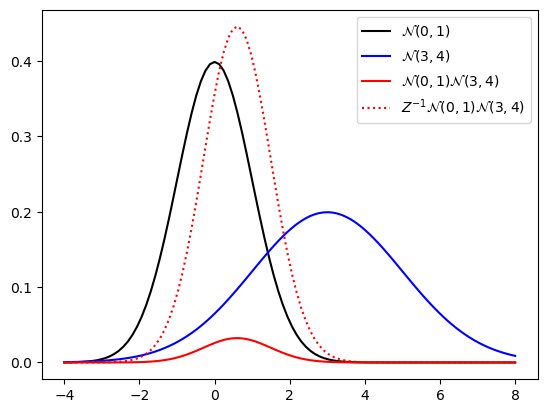The solid and dotted red curves are identical up to a scaling factor $Z$.

### Bayesian Inference with multiple Observations¶

• Now consider that we measure a data set $D = \{x_1, x_2, \ldots, x_N\}$, with measurements \begin{align*} x_n &= \theta + \epsilon_n \\ \epsilon_n &\sim \mathcal{N}(0,\sigma^2) \end{align*} and the same prior for $\theta$: \begin{align*} \theta &\sim \mathcal{N}(\mu_0,\sigma_0^2) \\ \end{align*}

• Let's derive a distribution for the next sample $x_{N+1}$.

##### inference¶
• Clearly, the posterior for $\theta$ is now \begin{align*} p(\theta|D) \propto \underbrace{\mathcal{N}(\theta|\mu_0,\sigma_0^2)}_{\text{prior}} \cdot \underbrace{\prod_{n=1}^N \mathcal{N}(x_n|\theta,\sigma^2)}_{\text{likelihood}} \end{align*} which is a multiplication of $N+1$ Gaussians and is therefore also Gaussian distributed.
• Using the property that precisions and precision-weighted means add when Gaussians are multiplied, we can immediately write the posterior $$p(\theta|D) = \mathcal{N} (\theta |\, \mu_N, \sigma_N^2)$$ as \begin{align*} \frac{1}{\sigma_N^2} &= \frac{1}{\sigma_0^2} + \sum_n \frac{1}{\sigma^2} \tag{B-2.142}\\ \mu_N &= \sigma_N^2 \, \left( \frac{1}{\sigma_0^2} \mu_0 + \sum_n \frac{1}{\sigma^2} x_n \right) \tag{B-2.141} \end{align*}
##### application: prediction of future sample¶
• We now have a posterior for the model parameters. Let's write down what we know about the next sample $x_{N+1}$.
\begin{align*} p(x_{N+1}|D_N) &= \int p(x_{N+1}|\theta) p(\theta|D_{N})\mathrm{d}\theta \\ &= \int \mathcal{N}(x_{N+1}|\theta,\sigma^2) \mathcal{N}(\theta|\mu_N,\sigma^2_N) \mathrm{d}\theta \\ &= \mathcal{N}(x_{N+1}|\mu_N, \sigma^2_N +\sigma^2 ) \tag{use SRG-6} \end{align*}
• Uncertainty about $x_{N+1}$ comprises both uncertainty about the parameter ($\sigma_N^2$) and observation noise $\sigma^2$.

### Maximum Likelihood Estimation for the Gaussian¶

• In order to determine the maximum likelihood estimate of $\theta$, we let $\sigma_0^2 \rightarrow \infty$ (leads to uniform prior for $\theta$), yielding $\frac{1}{\sigma_N^2} = \frac{N}{\sigma^2}$ and consequently \begin{align*} \mu_{\text{ML}} = \left.\mu_N\right\vert_{\sigma_0^2 \rightarrow \infty} = \sigma_N^2 \, \left( \frac{1}{\sigma^2}\sum_n x_n \right) = \frac{1}{N} \sum_{n=1}^N x_n \end{align*}
• As expected, having an expression for the maximum likelihood estimate, it is now possible to rewrite the (Bayesian) posterior mean for $\theta$ as (exercise) \begin{align*} \underbrace{\mu_N}_{\text{posterior}} &= \frac{\sigma^2}{N} \, \left( \frac{1}{\sigma_0^2} \mu_0 + \sum_n \frac{1}{\sigma^2} x_n \right) \\ &= \underbrace{\mu_0}_{\text{prior}} + \underbrace{\underbrace{\frac{N \sigma_0^2}{N \sigma_0^2 + \sigma^2}}_{\text{gain}}\cdot \underbrace{\left(\mu_{\text{ML}} - \mu_0 \right)}_{\text{prediction error}}}_{\text{correction}}\tag{B-2.141} \end{align*}
• Hence, the posterior mean always lies somewhere between the prior mean $\mu_0$ and the maximum likelihood estimate (the "data" mean) $\mu_{\text{ML}}$.

### Conditioning and Marginalization of a Gaussian¶

• Let $z = \begin{bmatrix} x \\ y \end{bmatrix}$ be jointly normal distributed as
\begin{align*} p(z) &= \mathcal{N}(z | \mu, \Sigma) =\mathcal{N} \left( \begin{bmatrix} x \\ y \end{bmatrix} \left| \begin{bmatrix} \mu_x \\ \mu_y \end{bmatrix}, \begin{bmatrix} \Sigma_x & \Sigma_{xy} \\ \Sigma_{yx} & \Sigma_y \end{bmatrix} \right. \right) \end{align*}
• Since covariance matrices are by definition symmetric, it follows that $\Sigma_x$ and $\Sigma_y$ are symmetric and $\Sigma_{xy} = \Sigma_{yx}^T$.
• Let's factorize $p(z) = p(x,y)$ into $p(y|x)\cdot p(x)$ through conditioning and marginalization.
$$\begin{equation*} \text{conditioning: }\boxed{ p(y|x) = \mathcal{N}\left(y\,|\,\mu_y + \Sigma_{yx}\Sigma_x^{-1}(x-\mu_x),\, \Sigma_y - \Sigma_{yx}\Sigma_x^{-1}\Sigma_{xy} \right)} \end{equation*}$$
$$\begin{equation*} \text{marginalization: } \boxed{ p(x) = \mathcal{N}\left( x|\mu_x, \Sigma_x \right)} \end{equation*}$$
• proof: in Bishop pp.87-89
• Hence, conditioning and marginalization in Gaussians leads to Gaussians again. This is very useful for applications to Bayesian inference in jointly Gaussian systems.
• With a natural parameterization of the Gaussian $p(z) = \mathcal{N}_c(z|\eta,\Lambda)$ with precision matrix $\Lambda = \Sigma^{-1} = \begin{bmatrix} \Lambda_x & \Lambda_{xy} \\ \Lambda_{yx} & \Lambda_y \end{bmatrix}$, the conditioning operation results in a simpler result, see Bishop pg.90, eqs. 2.96 and 2.97.
• As an exercise, interpret the formula for the conditional mean ($\mathbb{E}[y|x]=\mu_y + \Sigma_{yx}\Sigma_x^{-1}(x-\mu_x)$) as a prediction-correction operation.

### Code Example: Joint, Marginal, and Conditional Gaussian Distributions¶

• Let's plot of the joint, marginal, and conditional distributions.
In :
using PyPlot, Distributions

μ = [1.0; 2.0]
Σ = [0.3 0.7;
0.7 2.0]
joint = MvNormal(μ,Σ)
marginal_x = Normal(μ, sqrt(Σ[1,1]))

#Plot p(x,y)
subplot(221)
x_range = y_range = range(-2,stop=5,length=1000)
joint_pdf = [ pdf(joint, [x_range[i];y_range[j]]) for  j=1:length(y_range), i=1:length(x_range)]
imshow(joint_pdf, origin="lower", extent=[x_range, x_range[end], y_range, y_range[end]])
grid(); xlabel("x"); ylabel("y"); title("p(x,y)"); tight_layout()

# Plot p(x)
subplot(222)
plot(range(-2,stop=5,length=1000), pdf.(marginal_x, range(-2,stop=5,length=1000)))
grid(); xlabel("x"); ylabel("p(x)"); title("Marginal distribution p(x)"); tight_layout()

# Plot p(y|x)
x = 0.1
conditional_y_m = μ+Σ[2,1]*inv(Σ[1,1])*(x-μ)
conditional_y_s2 = Σ[2,2] - Σ[2,1]*inv(Σ[1,1])*Σ[1,2]
conditional_y = Normal(conditional_y_m, sqrt.(conditional_y_s2))
subplot(223)
plot(range(-2,stop=5,length=1000), pdf.(conditional_y, range(-2,stop=5,length=1000)))
grid(); xlabel("y"); ylabel("p(y|x)"); title("Conditional distribution p(y|x)"); tight_layout()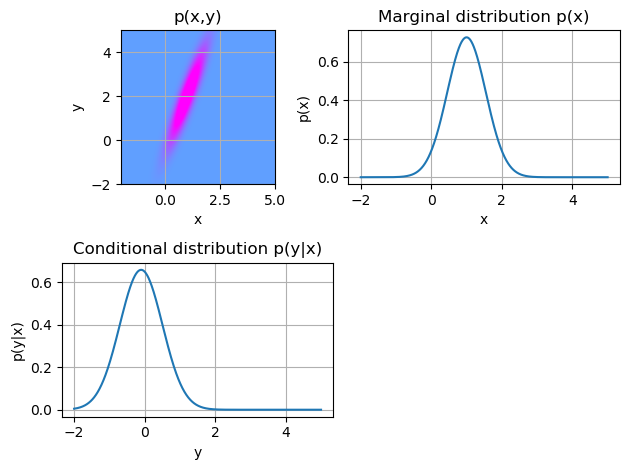As is clear from the plots, the conditional distribution is a renormalized slice from the joint distribution.

### Example: Conditioning of Gaussian¶

• Consider (again) the system \begin{align*} p(x\,|\,\theta) &= \mathcal{N}(x\,|\,\theta,\sigma^2) \end{align*} with a Gaussian prior for $\theta$: $$p(\theta) = \mathcal{N}(\theta\,|\,\mu_0,\sigma_0^2)$$
• Let $z = \begin{bmatrix} x \\ \theta \end{bmatrix}$. The distribution for $z$ is then given by (Exercise)
$$p(z) = p\left(\begin{bmatrix} x \\ \theta \end{bmatrix}\right) = \mathcal{N} \left( \begin{bmatrix} x\\ \theta \end{bmatrix} \,\left|\, \begin{bmatrix} \mu_0\\ \mu_0\end{bmatrix}, \begin{bmatrix} \sigma_0^2+\sigma^2 & \sigma_0^2\\ \sigma_0^2 &\sigma_0^2 \end{bmatrix} \right. \right)$$
• Direct substitution of the rule for Gaussian conditioning leads to the posterior (derivation as an Exercise): \begin{align*} p(\theta|x) &= \mathcal{N} \left( \theta\,|\,\mu_1, \sigma_1^2 \right)\,, \end{align*} with \begin{align*} K &= \frac{\sigma_0^2}{\sigma_0^2+\sigma^2} \qquad \text{(K is called: Kalman gain)}\\ \mu_1 &= \mu_0 + K \cdot (x-\mu_0)\\ \sigma_1^2 &= \left( 1-K \right) \sigma_0^2 \end{align*}
• $\Rightarrow$ Moral: For jointly Gaussian systems, we can do inference simply in one step by using the formulas for conditioning and marginalization.

### Recursive Bayesian Estimation¶

• Consider the signal $x_t=\theta+\epsilon_t$, where $D_t= \left\{x_1,\ldots,x_t\right\}$ is observed sequentially (over time).

• Problem: Derive a recursive algorithm for $p(\theta|D_t)$, i.e., an update rule for (posterior) $p(\theta|D_t)$ based on (prior) $p(\theta|D_{t-1})$ and (new observation) $x_t$.

##### Model specification¶
• Let's define the estimate after $t$ observations (i.e., our solution ) as $p(\theta|D_t) = \mathcal{N}(\theta\,|\,\mu_t,\sigma_t^2)$.

• We define the joint distribution for $\theta$ and $x_t$, given background $D_{t-1}$, by

\begin{align*} p(x_t,\theta \,|\, D_{t-1}) &= p(x_t|\theta) \, p(\theta|D_{t-1}) \\ &= \underbrace{\mathcal{N}(x_t\,|\, \theta,\sigma^2)}_{\text{likelihood}} \, \underbrace{\mathcal{N}(\theta\,|\,\mu_{t-1},\sigma_{t-1}^2)}_{\text{prior}} \end{align*}
##### Inference¶
• Use Bayes rule, \begin{align*} p(\theta|D_t) &= p(\theta|x_t,D_{t-1}) \\ &\propto p(x_t,\theta | D_{t-1}) \\ &= p(x_t|\theta) \, p(\theta|D_{t-1}) \\ &= \mathcal{N}(x_t|\theta,\sigma^2) \, \mathcal{N}(\theta\,|\,\mu_{t-1},\sigma_{t-1}^2) \\ &= \mathcal{N}(\theta|x_t,\sigma^2) \, \mathcal{N}(\theta\,|\,\mu_{t-1},\sigma_{t-1}^2) \;\;\text{(note this trick)}\\ &= \mathcal{N}(\theta|\mu_t,\sigma_t^2) \;\;\text{(use Gaussian multiplication formula SRG-6)} \end{align*} with \begin{align*} K_t &= \frac{\sigma_{t-1}^2}{\sigma_{t-1}^2+\sigma^2} \qquad \text{(Kalman gain)}\\ \mu_t &= \mu_{t-1} + K_t \cdot (x_t-\mu_{t-1})\\ \sigma_t^2 &= \left( 1-K_t \right) \sigma_{t-1}^2 \end{align*}
• This linear sequential estimator of mean and variance in Gaussian observations is called a Kalman Filter.
• Note that the uncertainty about $\theta$ decreases over time (since $0<(1-K_t)<1$). Since we assume that the statistics of the system do not change (stationarity), each new sample provides new information.
• Recursive Bayesian estimation is the basis for adaptive signal processing algorithms such as Least Mean Squares (LMS) and Recursive Least Squares (RLS).

### Code Example: Kalman Filter¶

• Let's implement the Kalman filter described above. We'll use it to recursively estimate the value of $\theta$ based on noisy observations.
In :
using PyPlot

N = 50                         # Number of observations
θ = 2.0                        # True value of the variable we want to estimate
σ_ϵ2 = 0.25                    # Observation noise variance
x = sqrt(σ_ϵ2) * randn(N) .+ θ # Generate N noisy observations of θ

t = 0
μ = fill!(Vector{Float64}(undef,N), NaN)    # Means of p(θ|D) over time
σ_μ2 = fill!(Vector{Float64}(undef,N), NaN) # Variances of p(θ|D) over time

function performKalmanStep()
# Perform a Kalman filter step, update t, μ, σ_μ2
global t += 1
if t>1 # Use posterior from prev. step as prior
K = σ_μ2[t-1] / (σ_ϵ2 + σ_μ2[t-1]) # Kalman gain
μ[t] = μ[t-1] + K*(x[t] - μ[t-1])  # Update mean using (1)
σ_μ2[t] = σ_μ2[t-1] * (1.0-K)      # Update variance using (2)
elseif t==1 # Use prior
# Prior p(θ) = N(0,1000)
K = 1000.0 / (σ_ϵ2 + 1000.0) # Kalman gain
μ[t] = 0 + K*(x[t] - 0)      # Update mean using (1)
σ_μ2[t] = 1000 * (1.0-K)     # Update variance using (2)
end
end

while t<N
performKalmanStep()
end

# Plot the 'true' value of θ, noisy observations x, and the recursively updated posterior p(θ|D)
t = collect(1:N)
plot(t, θ*ones(N), "k--")
plot(t, x, "rx")
plot(t, μ, "b-")
fill_between(t, μ-sqrt.(σ_μ2), μ+sqrt.(σ_μ2), color="b", alpha=0.3)
legend([L"\theta", L"x[t]", L"\mu[t]"])
xlim((1, N)); xlabel(L"t"); grid()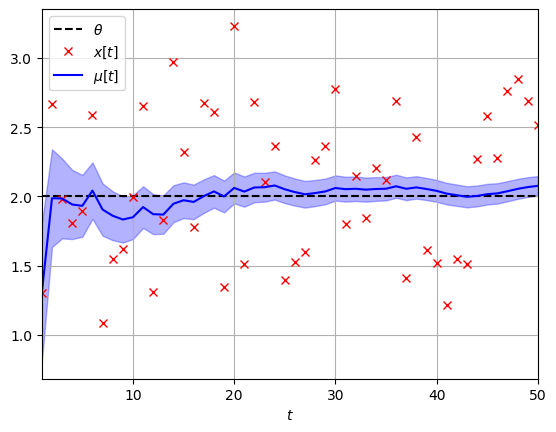The shaded area represents 2 standard deviations of posterior $p(\theta|D)$. The variance of the posterior is guaranteed to decrease monotonically for the standard Kalman filter.

### Product of Normally Distributed Variables¶

• (We've seen that) the sum of two Gausssian distributed variables is also Gaussian distributed.
• Has the product of two Gaussian distributed variables also a Gaussian distribution?
• No! In general this is a difficult computation. As an example, let's compute $p(z)$ for $Z=XY$ for the special case that $X\sim \mathcal{N}(0,1)$ and $Y\sim \mathcal{N}(0,1)$. \begin{align*} p(z) &= \int_{X,Y} p(z|x,y)\,p(x,y)\,\mathrm{d}x\mathrm{d}y \\ &= \frac{1}{2 \pi}\int \delta(z-xy) \, e^{-(x^2+y^2)/2} \, \mathrm{d}x\mathrm{d}y \\ &= \frac{1}{\pi} \int_0^\infty \frac{1}{x} e^{-(x^2+z^2/x^2)/2} \, \mathrm{d}x \\ &= \frac{1}{\pi} \mathrm{K}_0( \lvert z\rvert )\,. \end{align*} where $\mathrm{K}_n(z)$ is a modified Bessel function of the second kind.

### Code Example: Product of Gaussian Distributions¶

• We plot $p(Z=XY)$ and $p(X)p(Y)$ for $X\sim\mathcal{N}(0,1)$ and $Y \sim \mathcal{N}(0,1)$ to give an idea of how these distributions differ.
In :
using PyPlot, Distributions, SpecialFunctions
X = Normal(0,1)
Y = Normal(0,1)
pdf_product_std_normals(z::Vector) = (besselk.(0, abs.(z))./π)
range1 = collect(range(-4,stop=4,length=100))
plot(range1, pdf.(X, range1))
plot(range1, pdf.(X,range1).*pdf.(Y,range1))
plot(range1, pdf_product_std_normals(range1))
legend([L"p(X)=p(Y)=\mathcal{N}(0,1)", L"p(X)*p(Y)",L"p(Z=X*Y)"]); grid()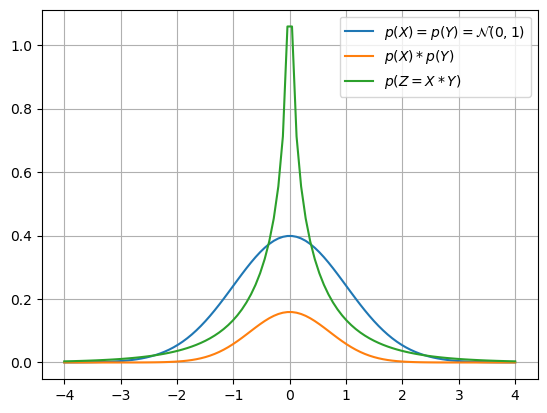• In short, Gaussian-distributed variables remain Gaussian in linear systems, but this is not the case in non-linear systems.

### Solution to Example Problem¶

We apply maximum likelihood estimation to fit a 2-dimensional Gaussian model ($m$) to data set $D$. Next, we evaluate $p(x_\bullet \in S | m)$ by (numerical) integration of the Gaussian pdf over $S$: $p(x_\bullet \in S | m) = \int_S p(x|m) \mathrm{d}x$.

In :
using HCubature, LinearAlgebra# Numerical integration package
# Maximum likelihood estimation of 2D Gaussian
N = length(sum(D,dims=1))
μ = 1/N * sum(D,dims=2)[:,1]
D_min_μ = D - repeat(μ, 1, N)
Σ = Hermitian(1/N * D_min_μ*D_min_μ')
m = MvNormal(μ, convert(Matrix, Σ));

# # Contour plot of estimated Gaussian density
A = Matrix{Float64}(undef,100,100); B = Matrix{Float64}(undef,100,100)
density = Matrix{Float64}(undef,100,100)
for i=1:100
for j=1:100
A[i,j] = a = (i-1)*6/100 .- 2
B[i,j] = b = (j-1)*6/100 .- 3
density[i,j] = pdf(m, [a,b])
end
end
c = contour(A, B, density, 6, zorder=1)
PyPlot.set_cmap("cool")
clabel(c, inline=1, fontsize=10)

# Plot observations, x∙, and the countours of the estimated Gausian density
plotObservations(D)
plot(x_dot, x_dot, "ro")

# Numerical integration of p(x|m) over S:
(val,err) = hcubature((x)->pdf(m,x), [0., 1.], [2., 2.])
println("p(x⋅∈S|m) ≈ $(val)")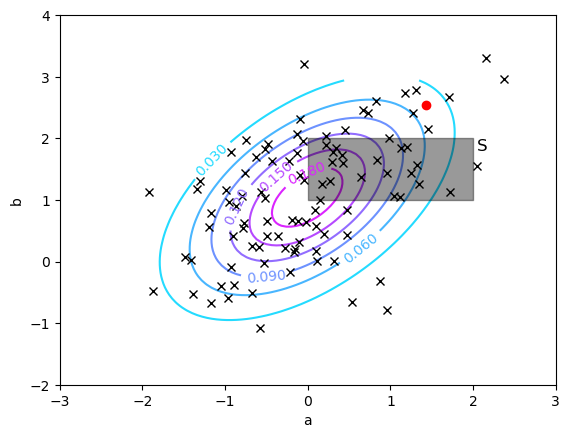p(x⋅∈S|m) ≈ 0.20063022272292289  ### Summary¶ • A linear transformation of a Gaussian-distributed (potentially multivariate) variable remains Gaussian. • Bayesian inference with Gaussian prior and likelihood leads to an analytically computable Gaussian posterior. • Here's a nice summary of Gaussian calculations by Sam Roweis. ## OPTIONAL SLIDES¶ ### Inference for the Precision Parameter of the Gaussian¶ • Again, we consider an observed data set$D = \{x_1, x_2, \ldots, x_N\}$and try to explain these data by a Gaussian distribution. • We discussed earlier Bayesian inference for the mean with a given variance. Now we will derive a posterior for the variance if the mean is given. (Technically, we will do the derivation for a precision parameter$\lambda = \sigma^{-2}, since the discussion is a bit more straightforward for the precision parameter). ##### model specification¶ • The likelihood for the precision parameter is \begin{align*} p(D|\lambda) &= \prod_{n=1}^N \mathcal{N}\left(x_n \,|\, \mu, \lambda^{-1} \right) \\ &\propto \lambda^{N/2} \exp\left\{ -\frac{\lambda}{2}\sum_{n=1}^N \left(x_n - \mu \right)^2\right\} \tag{B-2.145} \end{align*} • The conjugate distribution for this function of\lambda$is the Gamma distribution, given by $$p(\lambda\,|\,a,b) = \mathrm{Gam}\left( \lambda\,|\,a,b \right) \triangleq \frac{1}{\Gamma(a)} b^{a} \lambda^{a-1} \exp\left\{ -b \lambda\right\}\,, \tag{B-2.146}$$ where$a>0$and$b>0$are known as the shape and rate parameters, respectively.• (Bishop fig.2.13). Plots of the Gamma distribution$\mathrm{Gam}\left( \lambda\,|\,a,b \right) $for different values of$a$and$b$. • The mean and variance of the Gamma distribution evaluate to$\mathrm{E}\left( \lambda\right) = \frac{a}{b}$and$\mathrm{var}\left[\lambda\right] = \frac{a}{b^2}$. ##### inference¶ • We will consider a prior$p(\lambda) = \mathrm{Gam}\left( \lambda\,|\,a_0, b_0\right), which leads by Bayes rule to the posterior \begin{align*} p(\lambda\,|\,D) &\propto \underbrace{\lambda^{N/2} \exp\left\{ -\frac{\lambda}{2}\sum_{n=1}^N \left(x_n - \mu \right)^2\right\} }_{\text{likelihood}} \cdot \underbrace{\frac{1}{\Gamma(a_0)} b_0^{a_0} \lambda^{a_0-1} \exp\left\{ -b_0 \lambda\right\}}_{\text{prior}} \\ &\propto \mathrm{Gam}\left( \lambda\,|\,a_N,b_N \right) \end{align*} with \begin{align*} a_N &= a_0 + \frac{N}{2} \tag{B-2.150} \\ b_N &= b_0 + \frac{1}{2}\sum_n \left( x_n-\mu\right)^2 \tag{B-2.151} \end{align*} • Hence the posterior is again a Gamma distribution. By inspection of B-2.150 and B-2.151, we deduce that we can interpret2a_0$as the number of a priori (pseudo-)observations. • Since the most uninformative prior is given by$a_0=b_0 \rightarrow 0$, we can derive the maximum likelihood estimate for the precision as $$\lambda_{\text{ML}} = \left.\mathrm{E}\left[ \lambda\right]\right\vert_{a_0=b_0\rightarrow 0} = \left. \frac{a_N}{b_N}\right\vert_{a_0=b_0\rightarrow 0} = \frac{N}{\sum_{n=1}^N \left(x_n-\mu \right)^2}$$ • In short, if we do density estimation with a Gaussian distribution$\mathcal{N}\left(x_n\,|\,\mu,\sigma^2 \right)$for an observed data set$D = \{x_1, x_2, \ldots, x_N\}$, the maximum likelihood estimates for$\mu$and$\sigma^2\$ are given by \begin{align*} \mu_{\text{ML}} &= \frac{1}{N} \sum_{n=1}^N x_n \tag{B-2.121} \\ \sigma^2_{\text{ML}} &= \frac{1}{N} \sum_{n=1}^N \left(x_n - \mu_{\text{ML}} \right)^2 \tag{B-2.122} \end{align*}

• These estimates are also known as the sample mean and sample variance respectively.

In :
open("../../styles/aipstyle.html") do f